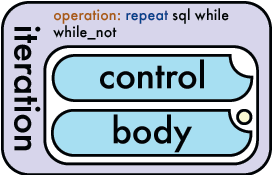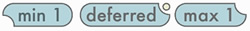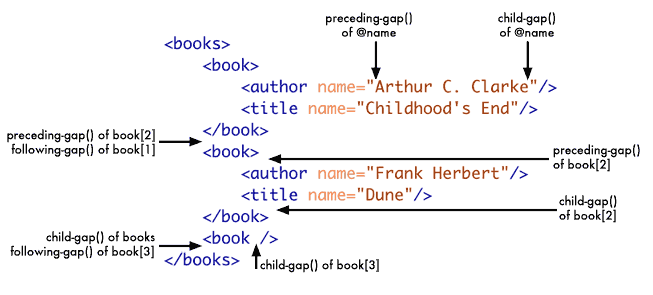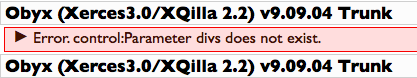### Object Iteration (Loop)

This tutorial introduces the iteration flow-function to create a loop

Following on from the "Loading a HTML Fragment" tutorial, we will now repeat the same process multiple times, in order to achieve this we will need an iteration (known in most programming languages as a loop), this is one of OBYX's four flow-functions.

Using the rational from previous tutorials we will call the XHTML file "iteration.ixml" and taking advantage of familiarity we use the same code.

``````iteration.ixml:
<!DOCTYPE html PUBLIC "-//W3C//DTD XHTML 1.0 Strict//EN" "http://www.w3.org/TR/xhtml1/DTD/xhtml1-strict.dtd">
<html xmlns="http://www.w3.org/1999/xhtml" xml:lang="en" lang="en">
<meta http-equiv="content-type" content="text/html; charset=utf-8" />
<title>Iteration </title>
<body>
<div></div>
</body>
</html>
``````

By now we should be treating the view on a "first name basis", as it is still the same as the previous tutorials, again the only changes are the title, page name and this time our div is empty, as this is where we are going to load our object using the iteration flow-function.

so now we have "iteration.ixml" as our view file

Below is the code to create our very simple object and we call it "xmlobject.xml", when we put this file in the same directory, it will get called by the OBYX file and output to the screen. In this tutorial we have the iteration that will modify the object and output the numbers from 1 to x where x is defined by the user.

``````xmlobject.xml:<?xml version="1.0" encoding="UTF-8"?>
<div xmlns="http://www.w3.org/1999/xhtml">This is a xml object</div>``````

Most of our OBYX control file is also identical to the file used in our loading a object tutorial, this time we call it "iteration.obyx" as iteration is the core flow-function for this program.

``<iteration note="iterate xml obejct 10 times and output to view">``The iteration element is used to strart the loop process. The iteration above is using the operation of repeat by default. The repeat operation loops code for a set number of times as defined by the control. As you may see in the diagram, repeat is the default operation as denoted by the blue text.

The control is an input like the element "input" in instruction. As such it can use all the attributes available to the "input" element. The control defines how many times the body input will be run.

We are now going to introduce a new concept of a "sysparm". A sysparm is a value that has been passed as a parameter from the URL or from a form. For example:

http://www.obyx.org/en/1/tutiteration.html?tutorial=great

Is passing the sysparm named tutorial to the server. When we access the sysparm in our OBYX file we get the value "great". Following on from this for our example we want to access the sysparm "divs". We do this in the following way:

``<control space="sysparm" value="divs" />``

Parameters passed via a url can have junk values that are of no use to OBYX. For example if we passed divs=20 as a parameter our code would work fine. On the other hand if we passed divs=x20 then our code would fail because we are expecting a number. We can take steps to reduce the likelyhood of our program failing by only using digits instead of all characters. We can do this by using the encoder attribute:

``<control space="sysparm" value="divs" encoder="digits" />``It is worth mentioning here that there is a defined structure for each input type within flow-functions. In many cases there must be a minimum or maximum of 1 occurrence on an element. The diagram on the right shows which elements on the OBYX map have min or max occurrences based on the type of notch they have in the upper right and lower left corners.

Using iteration as an example, we see that control and body can only have a maximum of one occurrence. Body also has a deferred status as denoted by the yellow circle. This means that it will not be run until the preceding input type has been fully evaluated.

The body is another input-type element. The body is executed x amount of times, where x is defined in the control. In our example code we are going to output a html fragment mulitple times based on a number provided by the user.

``````<body>
<instruction>
<output space="store" value="fragment#//h:div/text()" />
<input  space="field" value="#row"/>
</instruction>
<instruction>
<output space="store" value="view#//h:body/h:div/child-gap()" />
<input space="store" value="fragment" />
</instruction>
</body>
``````

When inside the body of an iteration we have access to special field types. One of these special fields is #row. #row returns the number of times that the body has been executed. #row always starts at 1.

As you may see there is a special xpath accessor not present in the xpath language. "child-gap()" tells OBYX to output the value at the end of an element. OBYX also has two other accessors, which are "preceding-gap()" and "following-gap()". As the names indicate OBYX uses these to output the values before and after the containing elements respectively. See diagram below.Below is the full OBYX prgram with the iteration code in it. Put the code below in a file called iteration.obyx. To call the code put the file in your webserver and call the url with ?divs=10 on the end. You should find that 10 divs labelled from 1 to 10 are output.

``````iteration.obyx:
<instruction xmlns="http://www.obyx.org">
<input>
<instruction note="declare namespace hook h for xhtml">
<output space="namespace" value="h"/>
<input value="http://www.w3.org/1999/xhtml"/>
</instruction>
<instruction note="load xml object into xhtml file">
<output space="store" value="view" />
<input space="file" value="iteration.ixml"/>
</instruction>
<instruction note="store object in a fragment">
<output space="store" value="fragment" />
<input space="file" value="xmlobject.xml" />
</instruction>
<iteration note="Output a div for number defined by sysparm 'divs'">
<control space="sysparm" value="divs" encoder="digits" />
<body>
<instruction>
<output space="store" value="fragment#//h:div/text()" />
<input  space="field" value="#row"/>
</instruction>
<instruction>
<output space="store" value="view#//h:body/h:div/child-gap()" />
<input space="store" value="fragment" />
</instruction>
</body>
</iteration>
<instruction note="load xml object into a xhtml file">
<input space="store" value="view" />
</instruction>
</input>
</instruction>``````
```<html xmlns="http://www.w3.org/1999/xhtml" lang="en" xml:lang="en">
<meta content="text/html; charset=utf-8"http-equiv="content-type"/>
<title>Iteration</title>
<body>
<div>
<div>1</div>
<div>2</div>
<div>3</div>
<div>4</div>
<div>5</div>
<div>6</div>
<div>7</div>
<div>8</div>
<div>9</div>
<div>10</div>
</div>
</body>
</html>```

As may of found out, if you leave out the parameter of "divs" from your URL we end up with an OBYX run-time error. The error will look similar to this:The error occurs because OBYX, unlike other scripting languages makes a clear distinction between a parameter/store/file existing and being empty. Tutorial 5: Validating User Input will go on to describe how to overcome this problem and also introduces how to write reusable OBYX functions.

### Summary of Iteration Tutorial

By the end of this tutorial you should have knowledge about the following concepts:

• The iteration flow-function including control and body inputs
• Encoding user input
• Input limits for flow-functions
• Special field type #row
• OBYX XPath accessors (child-gap(), following-gap() and preceding-gap())

Understanding these basic concepts are essential to OBYX programming. Spend time experimenting with each of the examples and ensure you understand why each of the elements are used.